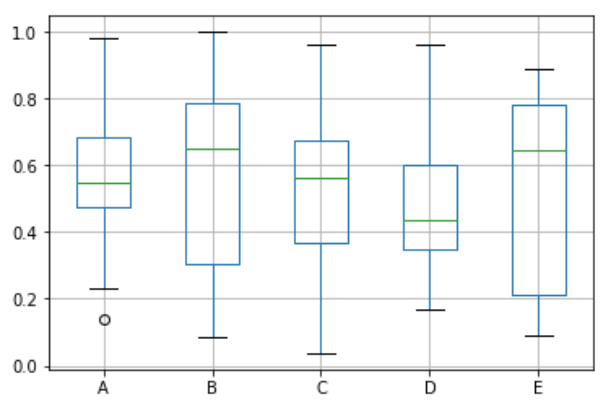# Python – Box Plots

Boxplots are a measure of how well distributed the data in a data set is. It divides the data set into three quartiles. This graph represents the minimum, maximum, median, first quartile and third quartile in the data set. It is also useful in comparing the distribution of data across data sets by drawing boxplots for each of them.

## Drawing a Box Plot

Boxplot can be drawn calling Series.box.plot() and DataFrame.box.plot(), or DataFrame.boxplot() to visualize the distribution of values within each column.

For instance, here is a boxplot representing five trials of 10 observations of a uniform random variable on [0,1).

```import pandas as pd
import numpy as np
df = pd.DataFrame(np.random.rand(10, 5), columns=['A', 'B', 'C', 'D', 'E'])
df.plot.box(grid='True')
```

Its output is as follows −